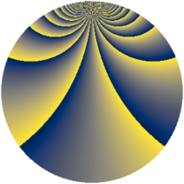# Properties

 Label 1152.3.bkLevel $1152$ Weight $3$ Character orbit 1152.bk Rep. character $\chi_{1152}(19,\cdot)$ Character field $\Q(\zeta_{32})$ Dimension $2544$ Sturm bound $576$

# Learn more

## Defining parameters

 Level: $$N$$ $$=$$ $$1152 = 2^{7} \cdot 3^{2}$$ Weight: $$k$$ $$=$$ $$3$$ Character orbit: $$[\chi]$$ $$=$$ 1152.bk (of order $$32$$ and degree $$16$$) Character conductor: $$\operatorname{cond}(\chi)$$ $$=$$ $$128$$ Character field: $$\Q(\zeta_{32})$$ Sturm bound: $$576$$

## Dimensions

The following table gives the dimensions of various subspaces of $$M_{3}(1152, [\chi])$$.

Total New Old
Modular forms 6208 2576 3632
Cusp forms 6080 2544 3536
Eisenstein series 128 32 96

## Trace form

 $$2544q + 16q^{2} - 16q^{4} + 16q^{5} - 16q^{7} + 16q^{8} + O(q^{10})$$ $$2544q + 16q^{2} - 16q^{4} + 16q^{5} - 16q^{7} + 16q^{8} - 16q^{10} + 16q^{11} - 16q^{13} + 16q^{14} - 16q^{16} + 16q^{17} - 16q^{19} + 16q^{20} - 16q^{22} + 16q^{23} - 16q^{25} + 16q^{26} - 16q^{28} + 16q^{29} - 16q^{31} + 16q^{32} - 16q^{34} + 16q^{35} - 16q^{37} + 16q^{38} - 16q^{40} + 16q^{41} - 16q^{43} + 16q^{44} - 16q^{46} + 16q^{47} - 16q^{49} - 608q^{50} + 1040q^{52} + 16q^{53} - 16q^{55} - 768q^{56} - 736q^{58} + 16q^{59} - 16q^{61} - 80q^{62} - 208q^{64} - 16q^{67} + 496q^{68} - 1360q^{70} + 16q^{71} - 16q^{73} + 1248q^{74} + 816q^{76} + 16q^{77} - 16q^{79} + 832q^{80} - 16q^{82} + 16q^{83} - 16q^{85} + 16q^{86} - 16q^{88} + 16q^{89} - 16q^{91} + 16q^{92} - 16q^{94} + 16q^{95} - 16q^{97} + 16q^{98} + O(q^{100})$$

## Decomposition of $$S_{3}^{\mathrm{new}}(1152, [\chi])$$ into newform subspaces

The newforms in this space have not yet been added to the LMFDB.

## Decomposition of $$S_{3}^{\mathrm{old}}(1152, [\chi])$$ into lower level spaces

$$S_{3}^{\mathrm{old}}(1152, [\chi]) \cong$$ $$S_{3}^{\mathrm{new}}(128, [\chi])$$$$^{\oplus 3}$$$$\oplus$$$$S_{3}^{\mathrm{new}}(384, [\chi])$$$$^{\oplus 2}$$